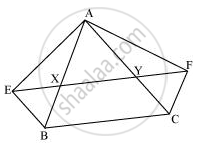# XY is a line parallel to side BC of a triangle ABC. If BE || AC and CF || AB meet XY at E and F respectively, show that ar (ABE) = ar (ACF) - Mathematics

XY is a line parallel to side BC of a triangle ABC. If BE || AC and CF || AB meet XY at E and F respectively, show that

ar (ABE) = ar (ACF)

#### SolutionIt is given that

XY || BC ⇒ EY || BC

BE || AC ⇒ BE || CY

Therefore, EBCY is a parallelogram.

It is given that

XY || BC ⇒ XF || BC

FC || AB ⇒ FC || XB

Therefore, BCFX is a parallelogram.

Parallelograms EBCY and BCFX are on the same base BC and between the same parallels BC and EF.

∴ Area (EBCY) = Area (BCFX) ... (1)

Consider parallelogram EBCY and ΔAEB

These lie on the same base BE and are between the same parallels BE and AC.

∴ Area (ΔABE) = 1/2Area (EBCY) ... (2)

Also, parallelogram BCFX and ΔACF are on the same base CF and between the same parallels CF and AB.

∴ Area (ΔACF) = 1/2Area (BCFX) ... (3)

From equations (1), (2), and (3), we obtain

Area (ΔABE) = Area (ΔACF)

Concept: Corollary: Triangles on the same base and between the same parallels are equal in area.
Is there an error in this question or solution?

#### APPEARS IN

NCERT Class 9 Maths
Chapter 9 Areas of Parallelograms and Triangles
Exercise 9.3 | Q 8 | Page 163